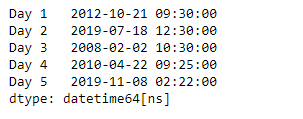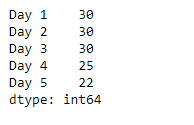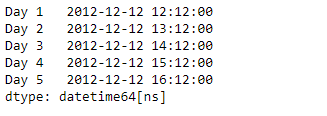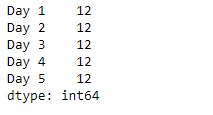Related Articles
Python | Pandas Series.dt.minute
• Last Updated : 20 Mar, 2019

`Series.dt` can be used to access the values of the series as datetimelike and return several properties. Pandas` Series.dt.minute` attribute return a numpy array containing the minutes of the datetime in the underlying data of the given series object.

Syntax: Series.dt.minute

Parameter : None

Returns : numpy array

Example #1: Use `Series.dt.minute` attribute to return the minutes of the datetime in the underlying data of the given Series object.

 `# importing pandas as pd ` `import` `pandas as pd ` ` `  `# Creating the Series ` `sr ``=` `pd.Series([``'2012-10-21 09:30'``, ``'2019-7-18 12:30'``, ``'2008-02-2 10:30'``, ` `               ``'2010-4-22 09:25'``, ``'2019-11-8 02:22'``]) ` ` `  `# Creating the index ` `idx ``=` `[``'Day 1'``, ``'Day 2'``, ``'Day 3'``, ``'Day 4'``, ``'Day 5'``] ` ` `  `# set the index ` `sr.index ``=` `idx ` ` `  `# Convert the underlying data to datetime  ` `sr ``=` `pd.to_datetime(sr) ` ` `  `# Print the series ` `print``(sr) `

Output :Now we will use `Series.dt.minute` attribute to return the minutes of the datetime in the underlying data of the given Series object.

 `# return the minutes ` `result ``=` `sr.dt.minute ` ` `  `# print the result ` `print``(result) `

Output :As we can see in the output, the `Series.dt.minute` attribute has successfully accessed and returned the minutes of the datetime in the underlying data of the given series object.

Example #2 : Use `Series.dt.minute` attribute to return the minutes of the datetime in the underlying data of the given Series object.

 `# importing pandas as pd ` `import` `pandas as pd ` ` `  `# Creating the Series ` `sr ``=` `pd.Series(pd.date_range(``'2012-12-12 12:12'``, ` `                       ``periods ``=` `5``, freq ``=` `'H'``)) ` ` `  `# Creating the index ` `idx ``=` `[``'Day 1'``, ``'Day 2'``, ``'Day 3'``, ``'Day 4'``, ``'Day 5'``] ` ` `  `# set the index ` `sr.index ``=` `idx ` ` `  `# Print the series ` `print``(sr) `

Output :Now we will use `Series.dt.minute` attribute to return the minutes of the datetime in the underlying data of the given Series object.

 `# return the minutes ` `result ``=` `sr.dt.minute ` ` `  `# print the result ` `print``(result) `

Output :As we can see in the output, the `Series.dt.minute` attribute has successfully accessed and returned the minutes of the datetime in the underlying data of the given series object.

Attention geek! Strengthen your foundations with the Python Programming Foundation Course and learn the basics.

To begin with, your interview preparations Enhance your Data Structures concepts with the Python DS Course.

My Personal Notes arrow_drop_up
Recommended Articles
Page :